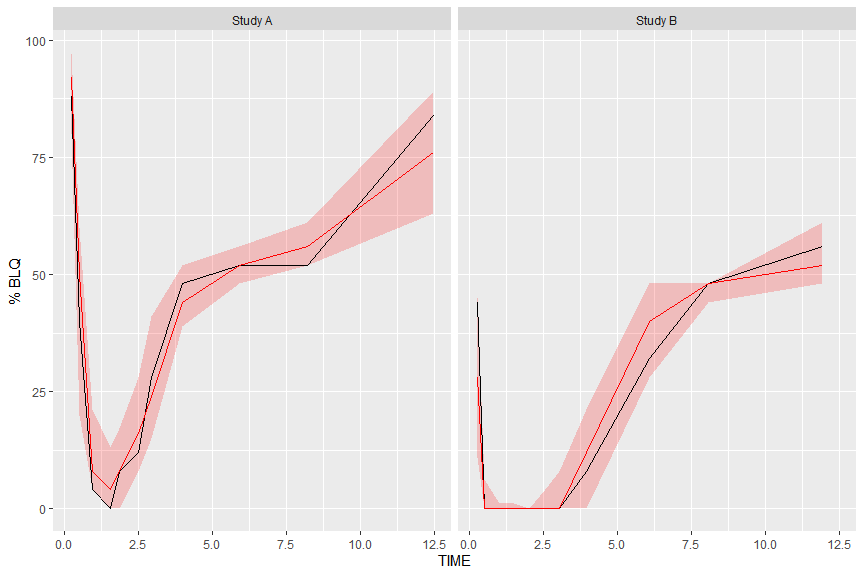Introduction to continuous VPC

Introduction

When deriving a Visual Predictive Check (VPC) you must:

• Have both observed and simulated datasets that include x and y variables, typically TIME and DV

• Compute prediction intervals on simulated versus observed data

When deriving a VPC you may want to:

• Stratify over variables in your model

• Censor data below LLOQ

• Perform prediction correction (pcVPC)

The tidyvpc package makes these steps fast and easy:

• By providing readable syntax using the %>% operator from magrittr.

• By using efficient backend computation, taking advantage of data.table parallelization.

• By providing traditional binning methods and new binless methods

• By using ggplot2 graphics engine to visualize the results of the VPC.

This document introduces you to the tidyvpc set of tools, and shows you how to apply them to tidyvpcobj to derive VPC.

All of the tidyvpc functions require the tidyvpcobj as the first argument, with the exception of the first function observed() in the piping chain, which takes a data.frame or data.table of the observed dataset. Rather than forcing the user to either save intermediate objects or nest functions, tidyvpc provides the %>% operator from magrittr. The result from one step is then “piped” into the next step, with the final function in the piping chain always vpcstats(). You can use the pipe to rewrite multiple operations that you can read left-to-right, top-to-bottom (reading the pipe operator as “then”).

Data

To explore the functionality of tidyvpc, we’ll use an altered version of obs_data(vpc::simple_data$obs) and sim_data(vpc::simple_data$sim) from the vpc package. These datasets contain all necessary variables to explore the functionality of tidyvpc including:

• DV (y variable)

• TIME (x variable)

• NTIME (nominal time for binning on x-variable)

• GENDER (gender variable for stratification, “M”, “F”)

• STUDY (study for stratification, “Study A”, “Study B”)

• PRED (prediction variable for pcVPC)

• MDV (Missing DV)

Data Structure

tidyvpc requires specific structure of observed and simulated data to successfully generate VPC.

See tidyvpc::obs_data and tidyvpc::sim_data for example data structure.

obs_data <- data.table::as.data.table(tidyvpc::obs_data)
#>    ID      TIME   DV AMT DOSE MDV NTIME GENDER   STUDY
#> 1:  1 0.0000000  0.0 150  150   1  0.00      M Study A
#> 2:  1 0.2157624 37.3   0  150   0  0.25      M Study A
#> 3:  1 0.4694366 62.2   0  150   0  0.50      M Study A
#> 4:  1 0.8271844 74.1   0  150   0  1.00      M Study A
#> 5:  1 1.7724895 75.1   0  150   0  1.50      M Study A
#> 6:  1 1.7142415 58.3   0  150   0  2.00      M Study A
sim_data <- data.table::as.data.table(tidyvpc::sim_data)
#>    ID REP      TIME     DV  IPRED   PRED AMT DOSE MDV NTIME
#> 1:  1   1 0.0000000  0.000  0.000  0.000 150  150   1  0.00
#> 2:  1   1 0.2157624 24.470 22.400 29.931   0  150   0  0.25
#> 3:  1   1 0.4694366 49.541 38.780 49.995   0  150   0  0.50
#> 4:  1   1 0.8271844 56.510 58.644 69.981   0  150   0  1.00
#> 5:  1   1 1.7724895 63.165 67.310 73.799   0  150   0  1.50
#> 6:  1   1 1.7142415 66.649 69.466 69.487   0  150   0  2.00

Preprocessing data

First, we’ll need to subset our data by filtering MDV == 0, which removes rows where both DV == 0 and TIME == 0.

obs_data <- obs_data[MDV == 0]
sim_data <- sim_data[MDV == 0]

Next, we’ll add the prediction variable from the first replicate of simulated data into our observed data.

obs_data$PRED <- sim_data[REP == 1, PRED] observed() The observed() function is always the first function used in the VPC piping chain and is used to specify the observed dataset and corresponding variables. There are three arguments that are required to use observed. The first argument is either a data.frame or data.table, the second argument is the name of x-variable in the observed data, and the third argument is the name of the y-variable. Note that variable names should be unquoted. library(tidyvpc) vpc <- observed(obs_data, x = TIME, y = DV) simulated() The simulated() function is used to specify the simulated dataset and corresponding variables. There are two arguments that are required in order to use simulated(). Since the function is “piped” in after the observed() function, the first argument is the tidyvpcobj and should not be included, followed by the name of the simulated data, then the name of y-variable in the simulated data. Variable names should be unquoted and x-variable should not be included as it is recycled from the observed() function. vpc <- observed(obs_data, x = TIME, y = DV) %>% simulated(sim_data, y = DV) binning() The binning() function provides the binning method to derive the VPC and should be inputted as a character string in the bin argument. Binning methods include: “ntile”, “pam”, “sd”, “equal”, “pretty”, “quantile”, “kmeans”, “jenks”, “centers”, “breaks”. Some methods such as “ntile” and “pam” will require you to specify the number of bins using the nbins argument (e.g., nbins = 9). If using bin = "centers" or bin = "breaks, you must also provide the centers/breaks argument as a numeric vector in the function (e.g., centers = c(1,3,5,7)). You can also bin directly on x-variable. If using this type of binning, the bin argument should be the unquoted variable name that you used in the observed() function (e.g., bin = NTIME for the Nominal Time variable in the data). Binning on x-variable, NTIME vpc <- observed(obs_data, x=TIME, y=DV) %>% simulated(sim_data, y=DV) %>% binning(bin = NTIME) Binning with “ntile” vpc <- observed(obs_data, x = TIME, y = DV) %>% simulated(sim_data, y = DV) %>% binning(bin = "ntile", nbins = 9) Binning with “breaks” vpc <- observed(obs_data, x = TIME, y = DV) %>% simulated(sim_data, y = DV) %>% binning(bin = "breaks", breaks = c(1,5,7,9,10)) binless() Note: The binless() examples below relate to a continuous VPC. See examples of binless() implementation for categorical VPC. Binless methods for continuous VPC use Additive Quantile Regression (AQR) in place of traditional binning. By default, binless() performs AQR at the 5%, 50%, and 95% quantiles, but you can change this using the qpred argument in vpcstats(), which takes a numeric vector of length 3 (e.g., qpred = c(.1, .5, .9) for the 10%, 50%, 90% quantiles). The lambda smoothing parameters for each quantile are optimized by default with AIC as indicated by the optimize = TRUE argument. If you would like to use different lambda values for each quantile set optimize = FALSE and specify lambda values for each quantile as a numeric vector of length 3 with the lambda argument (e.g., lambda = c(1,3,2) corresponds to the lambda values for the quantiles in the qpred argument (lower, median, upper)). Note: The higher the lambda value the smoother the fit to the data. Binless optimized vpc <- observed(obs_data, x=TIME, y=DV) %>% simulated(sim_data, y=DV) %>% binless() Binless with user-specified lambda values at 10%, 50%, 90% quantiles. Set optimize = FALSE and provide lambda smoothing parameters as a vector of length 3 for lower, median, upper quantiles. vpc <- observed(obs_data, x=TIME, y=DV) %>% simulated(sim_data, y=DV) %>% binless(qpred = c(0.1, 0.5, 0.9), optimize = FALSE, lambda = c(1,3,2)) vpcstats() The vpcstats() function is always the final function used in the piping chain and calculates the statistics needed to plot a VPC. If using binning()methods, you may specify alternative quantiles using the qpred argument. The default quantiles used are 5%, 50%, 95% (e.g., qpred = c(0.05, 0.5, 0.95)). vpc <- observed(obs_data, x=TIME, y=DV) %>% simulated(sim_data, y=DV) %>% binning(bin = NTIME) %>% vpcstats() plot(vpc)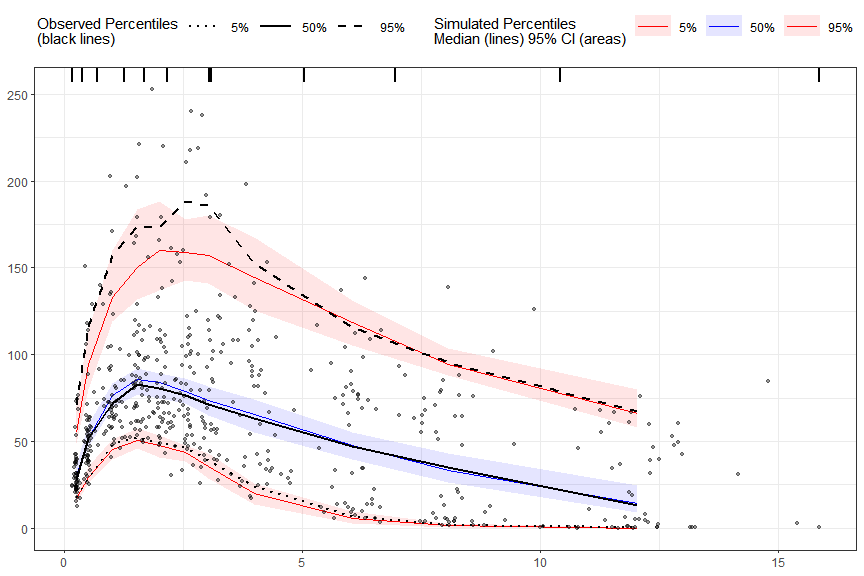stratify() To stratify VPC, include the stratify() function before using the binning() or binless() function and use the unquoted stratification variable(s) name as a formula. Let’s stratify on GENDER in the data, which contains 2 levels (GENDER = “M”, GENDER = “F”). Include as many stratification variables as your model calls for. vpc <- observed(obs_data, x=TIME, y=DV) %>% simulated(sim_data, y=DV) %>% stratify(~ GENDER) %>% binning(bin = "pam", nbins = 7) %>% vpcstats() plot(vpc)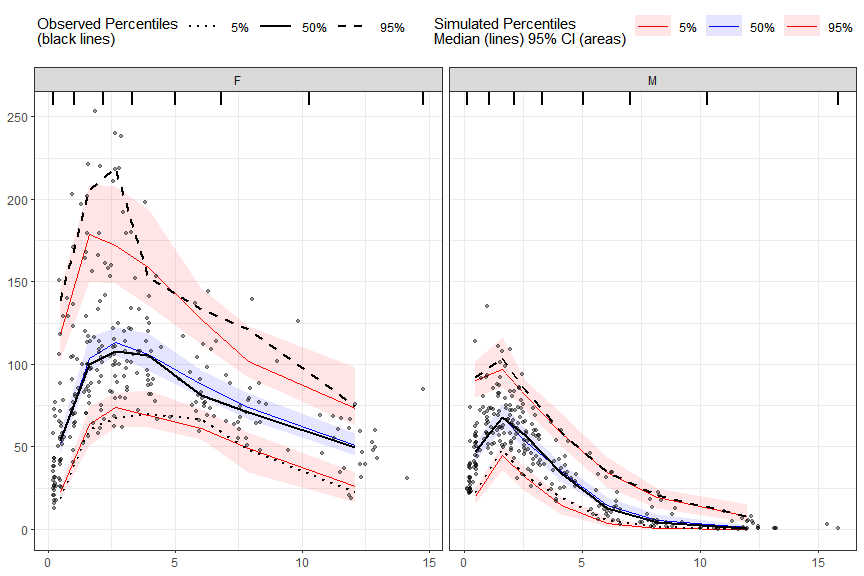Using multiple stratification variables GENDER and STUDY. vpc <- observed(obs_data, x=TIME, y=DV) %>% simulated(sim_data, y=DV) %>% stratify(~ GENDER + STUDY) %>% binless() %>% vpcstats() plot(vpc)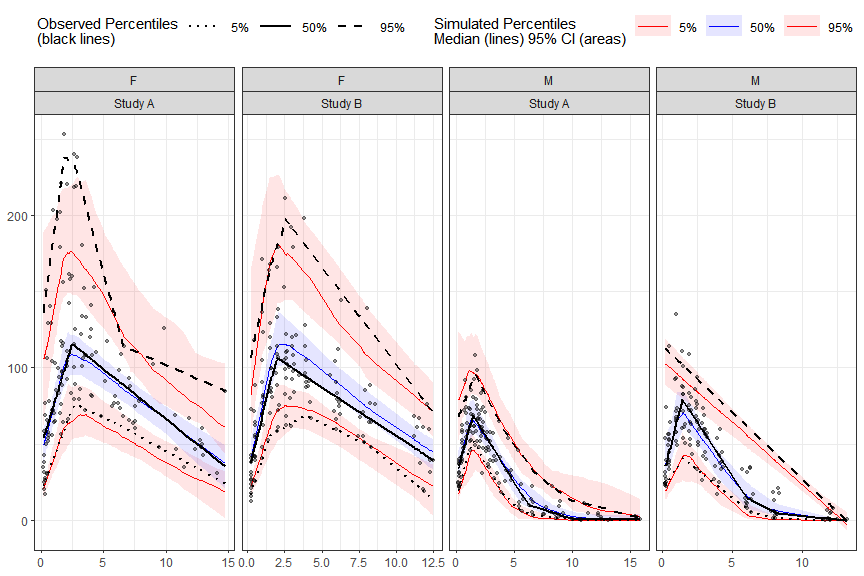censoring() Note: The censoring() function is only applicable for continuous VPC. To censor observed data below lower limit of quantification (LLOQ), include the censoring() function after simulated() and use the lloq argument to specify either a variable in the data or specific value for censoring. The blq argument creates a logical TRUE/FALSE in the data that indicates whether the value is below the limit of quantification and is typically defined as rows with DV < LLOQ in the data. Using the censoring() function will censor only observed data below lower limit of quantification when plotting, simulated data will still be plotted. Censoring using numeric value. vpc <- observed(obs_data, x=TIME, y=DV) %>% simulated(sim_data, y=DV) %>% censoring(blq=(DV < 25), lloq=25) %>% binning(bin = "jenks", nbins = 5) %>% vpcstats() plot(vpc)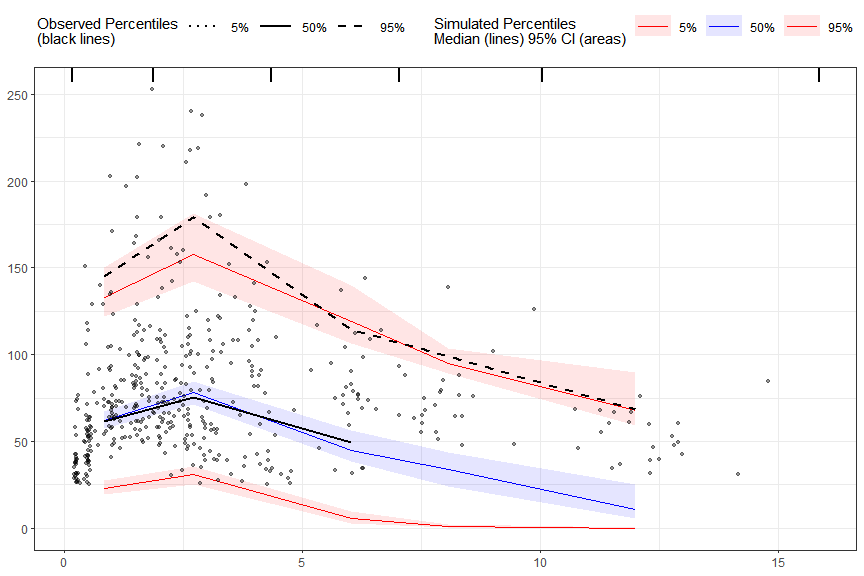Censoring using LLOQ variable in the data. First, let’s add an LLOQ variable to the observed data. obs_data$LLOQ <- 50

Then, we’ll specify lower limit of quantification as the unquoted variable name LLOQ in our data. Let’s also provide our own lambda values.

vpc <- observed(obs_data, x=TIME, y=DV) %>%
simulated(sim_data, y=DV) %>%
censoring(blq=(DV < LLOQ), lloq=LLOQ) %>%
binless(optimize = FALSE, lambda = c(1.5, 2.5, 1.7)) %>%
vpcstats()

plot(vpc)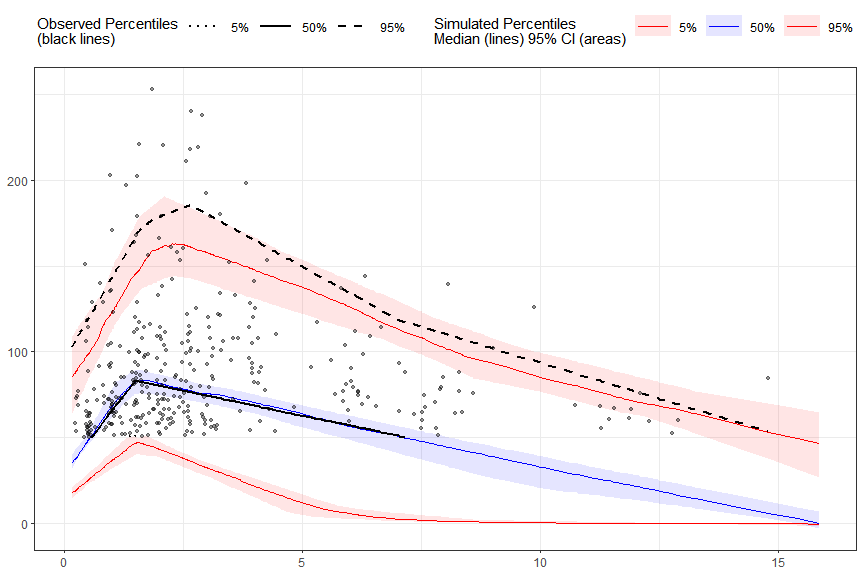The tidyvpc package also allows you to use different LLOQ for each level of stratification variable. We’ll set an LLOQ value of 50 for Study A and 25 for Study B and calculate statistics at 5%, 50%, 90% quantiles.

obs_data$LLOQ <- obs_data[, ifelse(STUDY == "Study A", 50, 25)] vpc <- observed(obs_data, x=TIME, y=DV) %>% simulated(sim_data, y=DV) %>% censoring(blq=(DV < LLOQ), lloq=LLOQ) %>% stratify(~ STUDY) %>% binning(bin = "pam", nbins = 4) %>% vpcstats(qpred = c(0.1, 0.5, 0.9)) plot(vpc)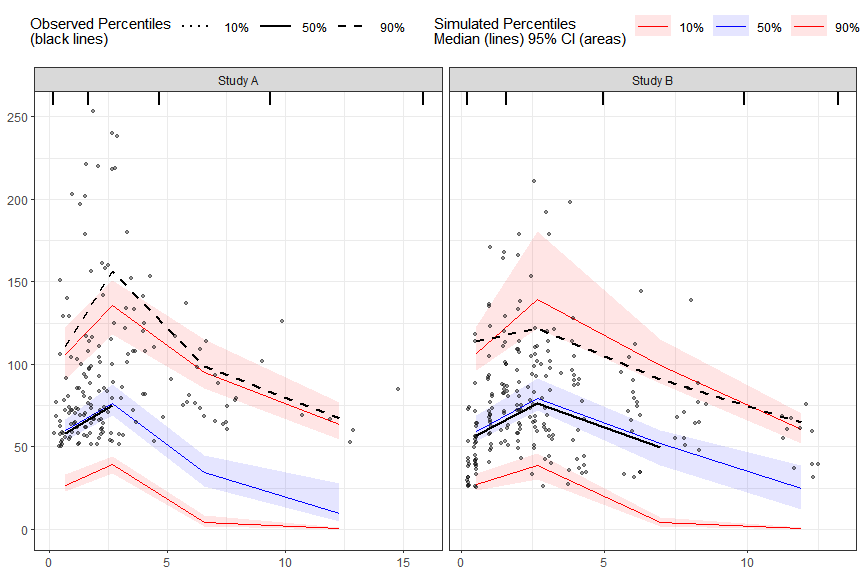predcorrect() Note: The predcorrect() function is only applicable for continuous VPC. To derive a prediction corrected VPC (pcVPC), use the predcorrect() function. The predcorrect() function takes one required argument, pred, which should be the unquoted variable name of the population prediction variable in the data. If using binning methods, the predcorrect() function should be called after binning(). If performing LOESS pcVPC for binless() methods, use predcorrect() before calling binless() and set binless(loess.ypc = TRUE). Note: If the model was fit using log scale of DV, make sure to include the argument log = TRUE in predcorrect() to perform the appropriate prediction correction calculation. Prediction corrected using binning methods. vpc <- observed(obs_data, x=TIME, y=DV) %>% simulated(sim_data, y=DV) %>% stratify(~GENDER) %>% binning(bin = NTIME) %>% predcorrect(pred=PRED) %>% vpcstats() plot(vpc)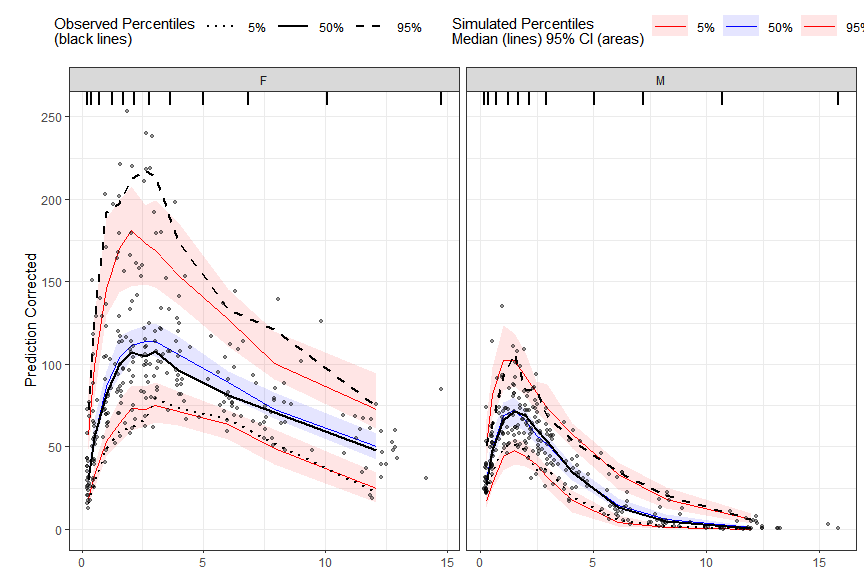LOESS prediction corrected using binless method for 10%, 50%, 90% quantiles. If optimize = TRUE, the LOESS smoothing parameter, span, will be automatically optimized using AIC. Note: predcorrect() must be called before binless() if setting loess.ypc = TRUE. vpc <- observed(obs_data, x=TIME, y=DV) %>% simulated(sim_data, y=DV) %>% stratify(~GENDER) %>% predcorrect(pred=PRED) %>% binless(qpred = c(0.1, 0.5, 0.9), optimize = TRUE, loess.ypc = TRUE) %>% vpcstats() plot(vpc)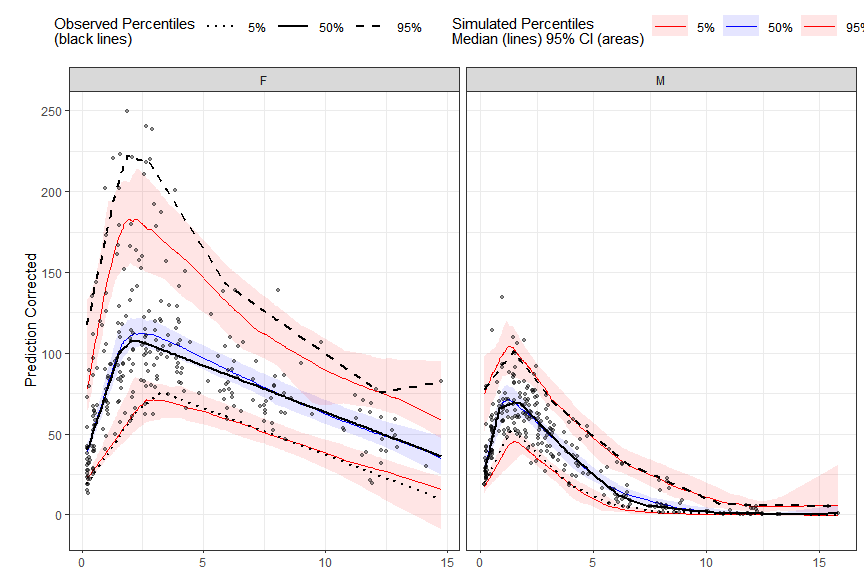To specify your own span value LOESS pcVPC instead of optimizing with AIC, use the span argument in the binless function. Span should be a numeric value between [0,1], with higher values providing a smoother fit. Remember, to also include the smoothing parameters for AQR by using the lambda argument and set optimize = FALSE. vpc <- observed(obs_data, x=TIME, y=DV) %>% simulated(sim_data, y=DV) %>% predcorrect(pred=PRED) %>% binless(optimize = FALSE, lambda = c(.95,3,1.2), loess.ypc = TRUE, span = .6) %>% vpcstats() plot(vpc)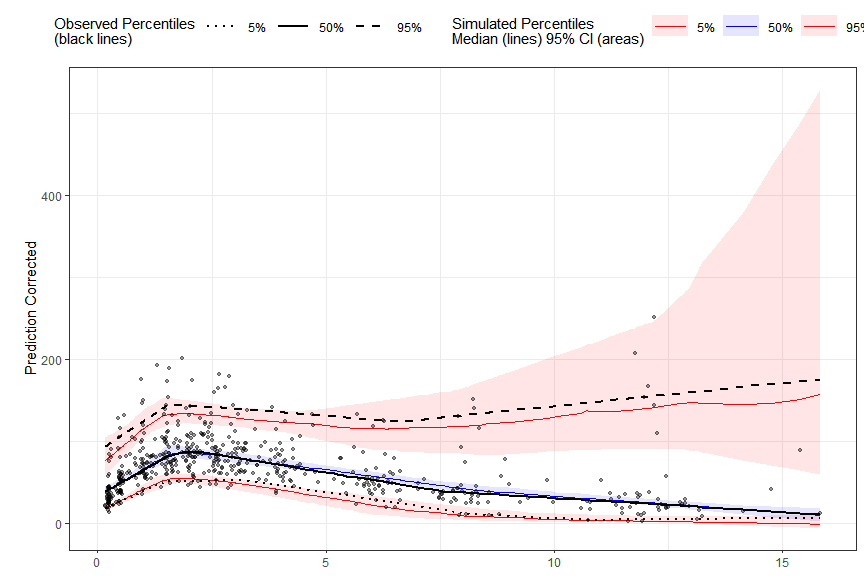Extending further Below are some examples of advanced use cases of the tidyvpc package. Different binning methods by strata To use different binning methods for different stratification variables, and/or for each level of stratification variable, use multiple calls to the binning() function in combination with the stratum argument. Make sure to set by.strata = T vpc <- observed(obs_data, x=TIME, y=DV) %>% simulated(sim_data, y=DV) %>% stratify(~ GENDER + STUDY) %>% binning(stratum = list(GENDER = "M", STUDY = "Study A"), bin = "jenks", nbins = 5, by.strata = T) %>% binning(stratum = list(GENDER = "F", STUDY = "Study A"), bin = "centers", centers = c(0.5,3,5,10,15), by.strata = T) %>% binning(stratum = list(GENDER = "M", STUDY = "Study B"), bin = "kmeans", by.strata = T) %>% binning(stratum = list(GENDER = "F", STUDY = "Study B"), bin = "pam", nbins = 5, by.strata = T) %>% predcorrect(pred=PRED) %>% vpcstats() plot(vpc)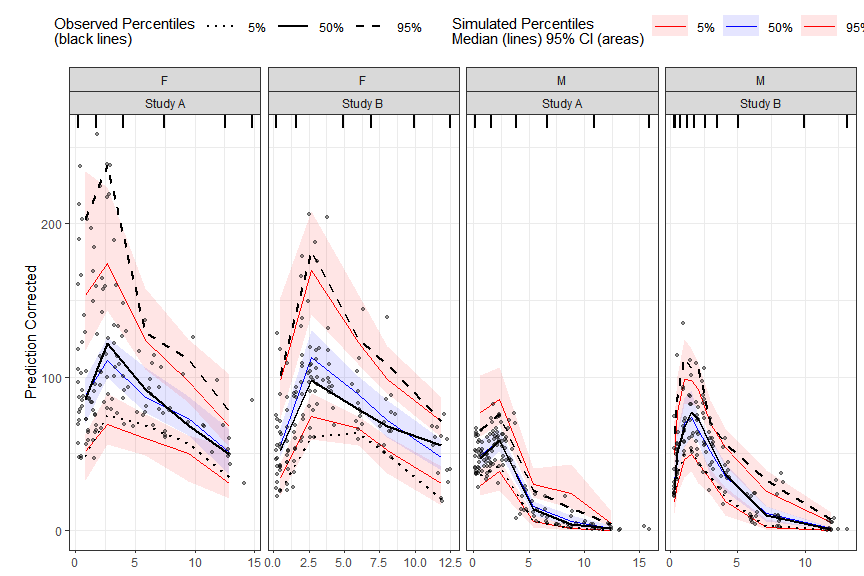Different smoothing values for each level of stratification variable To use different smoothing parameters for each level of stratification variable if using the binless() function, use a single call of the binless() function and include a data.frame with the column names of stratification variable and corresponding level. To use different span values for each level of stratification variable, use a vector the length of n levels of strata. Note: If using more than one stratification variable with the binless() function, you must set optimize = TRUE and optimize lambda and span using AIC. user_lambda <- data.frame(GENDER_F = c(2,4,2), GENDER_M = c(1.9,3,2.25) ) vpc <- observed(obs_data, x=TIME, y=DV) %>% simulated(sim_data, y=DV) %>% stratify(~ GENDER) %>% predcorrect(pred=PRED) %>% binless(optimize = FALSE, lambda = user_lambda, loess.ypc = TRUE, span = c(.6, .85)) %>% vpcstats(qpred = c(0.1, 0.5, 0.9)) plot(vpc)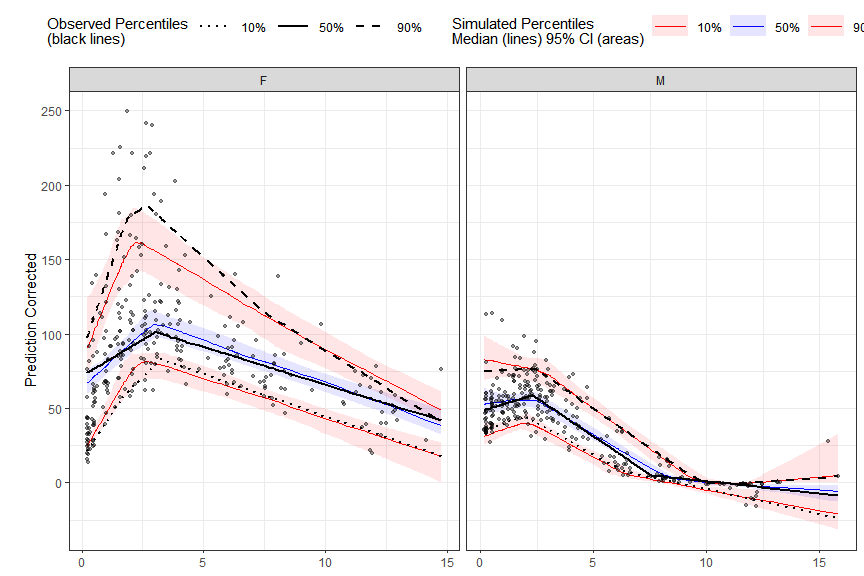Visualize bins If using binning() methods, you can visualize bins by using the plot() function on the tidyvpcobj without calling vpcstats(). Once you are satisfied with the binning method, simply call vpcstats() on the existing tidyvpcobj to compute VPC percentiles and prediction intervals (e.g., vpc %>% vpcstats()). vpc <- observed(obs_data, x=TIME, y=DV) %>% simulated(sim_data, y=DV) %>% binning(bin = "jenks", nbins = 7) plot(vpc)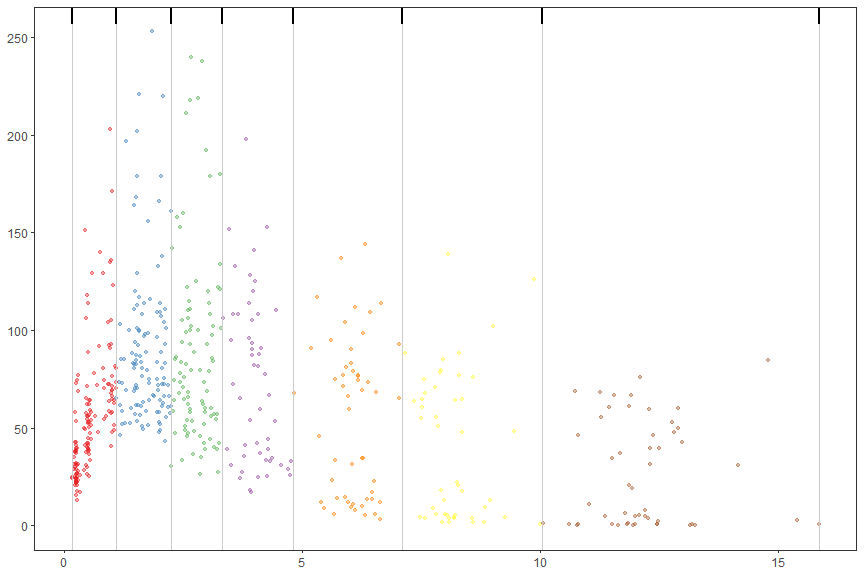Obtain bin information To obtain information about the bins, including the number of observations, xmedian, xmean, xmin, xmax, xmidpoint, xleft, xright, and xcenter, use the bininfo() function from tidyvpc. vpc <- observed(obs_data, x=TIME, y=DV) %>% simulated(sim_data, y=DV) %>% binning(bin = "jenks", nbins = 4) %>% vpcstats() bin_information <- bininfo(vpc) head(bin_information) #> bin nobs xmedian xmean xmin xmax xmid #> 1: [0.158,1.89) 213 0.8418133 0.8717959 0.1575342 1.860852 1.009193 #> 2: [1.89,4.83) 187 2.8021930 2.9458444 1.8851078 4.772347 3.328727 #> 3: [4.83,9.45) 96 6.6279698 6.9869973 4.8283449 9.259398 7.043872 #> 4: [9.45,15.8] 54 11.9639045 12.0388598 9.4470340 15.848161 12.647597 #> xleft xright xcenter #> 1: 0.1575342 1.872980 1.015257 #> 2: 1.8729798 4.800346 3.336663 #> 3: 4.8003458 9.353216 7.076781 #> 4: 9.3532162 15.848161 12.600688 Using ggplot2 with tidyvpc While the built-in plot() function make it easy to quickly visualize the derived VPC, the tidyvpcobj can be plotted using ggplot2 for complete plot customization. Plot VPC with ggplot2 library(ggplot2) obs_data$LLOQ <- obs_data[, ifelse(STUDY == "Study A", 50, 25)]

vpc <- observed(obs_data, x = TIME, y = DV) %>%
simulated(sim_data, y = DV) %>%
censoring(blq = DV < LLOQ, lloq = LLOQ) %>%
stratify(~STUDY) %>%
binning(bin = NTIME) %>%
vpcstats(qpred = c(0.1, 0.5, 0.9))

ggplot(vpc$stats, aes(x = xbin)) + facet_grid(~STUDY, scales = "free", as.table = FALSE) + geom_ribbon(aes(ymin = lo, ymax = hi, fill = qname, col = qname, group = qname),alpha = 0.1, col = NA) + geom_line(aes(y = md, col = qname, group = qname)) + geom_line(aes(y = y, linetype = qname), size = 1) + geom_hline(data=unique(obs_data[, .(STUDY, LLOQ)]), aes(yintercept=LLOQ), linetype="dotted", size=1) + geom_text(data = unique(vpc$data[, .(LLOQ), by = "STUDY"]),
aes(x = 10, y = LLOQ, label = paste("LLOQ", LLOQ, sep = "="), ), vjust = 1, hjust = 1) +
scale_colour_manual(name = "Simulated Percentiles\nMedian (lines) 95% CI (areas)",
breaks = c("q0.1", "q0.5", "q0.9"),
values = c("red", "blue", "red"),
labels = c("10%", "50%", "90%")) +
scale_fill_manual(name = "Simulated Percentiles\nMedian (lines) 95% CI (areas)",
breaks = c("q0.1", "q0.5", "q0.9"),
values = c("red", "blue", "red"),
labels = c("10%", "50%", "90%")) +
scale_linetype_manual(name = "Observed Percentiles\nMedian (lines) 95% CI (areas)",
breaks = c("q0.1", "q0.5", "q0.9"),
values = c("dotted", "solid", "dashed"),
labels = c("10%", "50%", "90%")) +
guides(fill = guide_legend(order = 2), colour = guide_legend(order = 2), linetype = guide_legend(order = 1)) +
theme(legend.position = "top", legend.key.width = grid::unit(1, "cm")) +
labs(x = "TIME", y = "Concentration") +
geom_point(data = vpc$obs, aes(x = x, y = y), size = 1, alpha = 0.1, show.legend = FALSE) + geom_vline(data = bininfo(vpc)[, .(x = sort(unique(c(xleft, xright)))), by = names(vpc$strat)],aes(xintercept = x), size = rel(0.5), col = "gray80") +
theme(panel.grid = element_blank()) +
geom_rug(data = bininfo(vpc)[, .(x = sort(unique(c(xleft, xright)))), by = names(vpc$strat)],aes(x = x), sides = "t", size = 1)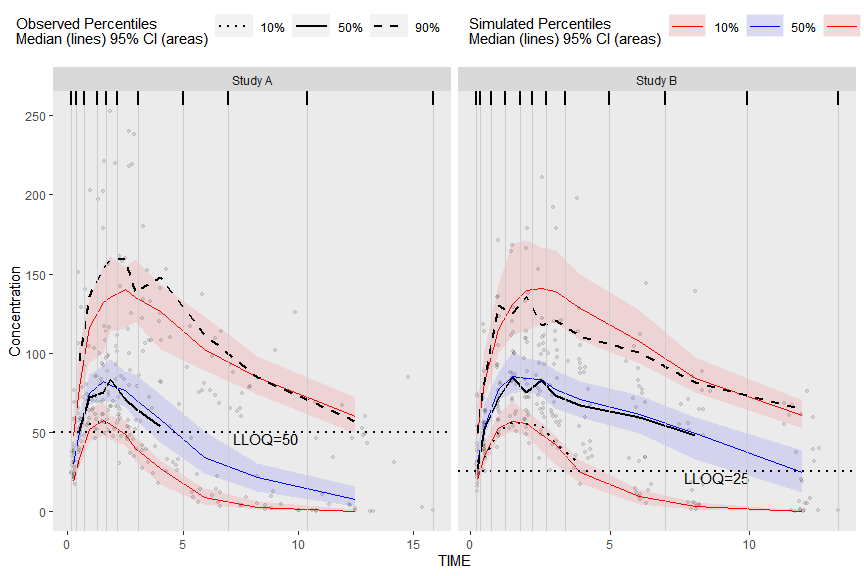Plot rectangles using bininfo() The results from bininfo() make it easy to plot a rectangle VPC using ggplot2. vpc <- observed(obs_data, x=TIME, y=DV) %>% simulated(sim_data, y=DV) %>% binning(bin = "jenks", nbins = 4) %>% vpcstats() #Get vpcstats df stats <- vpc$stats
#Get bininfo df
bin_information <- bininfo(vpc)
#Left join bin_info to vpcstats on bin
bin_information <- stats[bin_information, on = "bin"]
#Generate ymin
bin_information <- bin_information[, ymin := min(y), by = "bin"]
#Generate ymax
bin_information <- bin_information[, ymax := max(y), by = "bin"]
#>             bin      xbin qname      y        lo       md        hi nobs
#> 1: [0.158,1.89) 0.8418133 q0.05  22.94  19.58905  22.7307  27.73307  213
#> 2: [0.158,1.89) 0.8418133  q0.5  61.50  57.45770  61.7670  67.05757  213
#> 3: [0.158,1.89) 0.8418133 q0.95 144.40 121.90995 132.7460 149.47290  213
#> 4:  [1.89,4.83) 2.8021930 q0.05  29.38  21.34827  28.0135  32.11742  187
#> 5:  [1.89,4.83) 2.8021930  q0.5  74.10  70.76337  76.1745  82.59877  187
#> 6:  [1.89,4.83) 2.8021930 q0.95 179.00 141.91740 157.6140 181.46822  187
#>      xmedian     xmean      xmin     xmax     xmid     xleft   xright  xcenter
#> 1: 0.8418133 0.8717959 0.1575342 1.860852 1.009193 0.1575342 1.872980 1.015257
#> 2: 0.8418133 0.8717959 0.1575342 1.860852 1.009193 0.1575342 1.872980 1.015257
#> 3: 0.8418133 0.8717959 0.1575342 1.860852 1.009193 0.1575342 1.872980 1.015257
#> 4: 2.8021930 2.9458444 1.8851078 4.772347 3.328727 1.8729798 4.800346 3.336663
#> 5: 2.8021930 2.9458444 1.8851078 4.772347 3.328727 1.8729798 4.800346 3.336663
#> 6: 2.8021930 2.9458444 1.8851078 4.772347 3.328727 1.8729798 4.800346 3.336663
#>     ymin  ymax
#> 1: 22.94 144.4
#> 2: 22.94 144.4
#> 3: 22.94 144.4
#> 4: 29.38 179.0
#> 5: 29.38 179.0
#> 6: 29.38 179.0

Plot rectangles using ymin and ymax, the min/max y values in vpc$stats grouped by bin. ggplot(bin_information, aes(x = xbin)) + geom_line(aes(y = md, col = qname, group = qname)) + geom_line(aes(y = y, linetype = qname), size = 1) + geom_rect(aes(xmin= xleft,xmax= xright, ymin = ymin, ymax = ymax),alpha = .1, col = "black", fill = "green") + geom_point(data = vpc$obs, aes(x = x, y = y), size = 1, alpha = 0.1, show.legend = FALSE) +
scale_colour_manual(name = "Simulated Percentiles\nMedian (lines) 95% CI (areas)",
breaks = c("q0.05", "q0.5", "q0.95"),
values = c("red", "blue", "red"),
labels = c("5%", "50%", "95%")) +
scale_linetype_manual(name = "Observed Percentiles\nMedian (lines) 95% CI (areas)",
breaks = c("q0.05", "q0.5", "q0.95"),
values = c("dotted", "solid", "dashed"),
labels = c("5%", "50%", "95%")) +
geom_vline(data = bin_information[, .(x = sort(unique(c(xleft, xright))))],aes(xintercept = x), size = rel(0.5), col = "gray80") +
geom_rug(data = bin_information[, .(x = sort(unique(c(xleft, xright))))],aes(x = x), sides = "t", size = 1) +
guides(fill = guide_legend(order = 2), colour = guide_legend(order = 2), linetype = guide_legend(order = 1)) +
theme(legend.position = "top", legend.key.width = grid::unit(1, "cm")) +
labs(x = "TIME", y = "Concentration")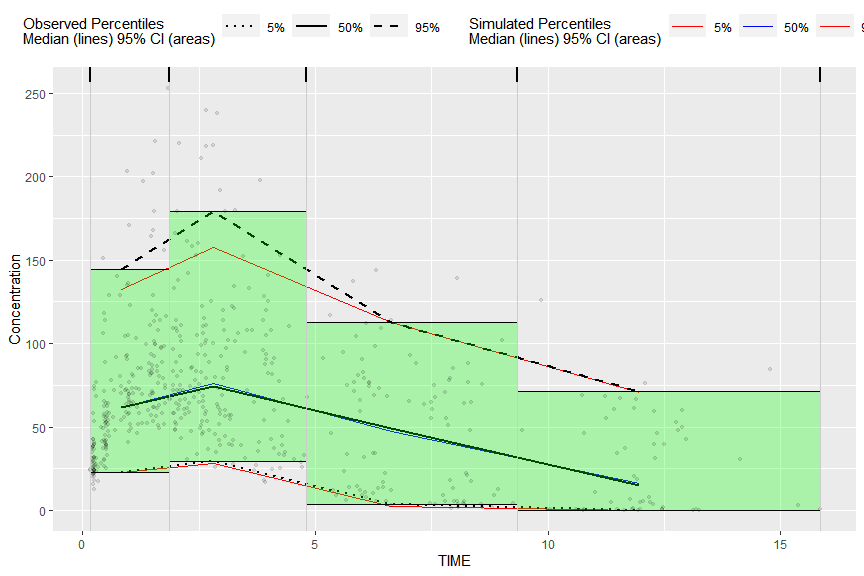Alternatively, we can obtain the required data for plotting used in the above bin_information data frame by merging vpc$stats and bininfo(vpc) on bin in the ggplot2 data argument. If stratifying, you will need to include the name of the stratification variable(s) in the data.table merge (e.g., vpc$stats[bininfo(vpc), on=c("STUDY", "bin")]). In the rectangle VPC below, we will stratify on STUDY and plot rectangles for each quantile.

obs_data$LLOQ <- obs_data[, ifelse(STUDY == "Study A", 50, 25)] vpc <- observed(obs_data, x = TIME, y = DV) %>% simulated(sim_data, y = DV) %>% censoring(blq = DV < LLOQ, lloq = LLOQ) %>% stratify(~STUDY) %>% binning(bin = NTIME) %>% vpcstats(qpred = c(0.1, 0.5, 0.9)) ggplot(vpc$stats[bininfo(vpc), on=c("STUDY", "bin")], aes(x = xbin)) +
facet_grid(~STUDY, scales = "free", as.table = FALSE) +
geom_rect(aes(xmin = xleft, xmax = xright, ymin = lo, ymax = hi, fill = qname, col = qname, group = qname),alpha = 0.1, col = NA) +
geom_segment(aes(x = xleft, xend = xright, y = md, yend = md, col = qname, group = qname)) +
geom_segment(aes(x = xleft, xend = xright, y = y, yend = y, linetype = qname), size = 1) +
geom_line(aes(y = md, col = qname, group = qname)) +
geom_line(aes(y = y, linetype = qname), size = 1) +
geom_hline(data=unique(obs_data[, .(STUDY, LLOQ)]), aes(yintercept=LLOQ), linetype="dotted", size=1) +
geom_text(data = unique(vpc$data[, .(LLOQ), by = "STUDY"]), aes(x = 10, y = LLOQ, label = paste("LLOQ", LLOQ, sep = "="), ), vjust = 1, hjust = 1) + scale_colour_manual(name = "Simulated Percentiles\nMedian (lines) 95% CI (areas)", breaks = c("q0.1", "q0.5", "q0.9"), values = c("red", "blue", "red"), labels = c("10%", "50%", "90%")) + scale_fill_manual(name = "Simulated Percentiles\nMedian (lines) 95% CI (areas)", breaks = c("q0.1", "q0.5", "q0.9"), values = c("red", "blue", "red"), labels = c("10%", "50%", "90%")) + scale_linetype_manual(name = "Observed Percentiles\nMedian (lines) 95% CI (areas)", breaks = c("q0.1", "q0.5", "q0.9"), values = c("dotted", "solid", "dashed"), labels = c("10%", "50%", "90%")) + guides(fill = guide_legend(order = 2), colour = guide_legend(order = 2), linetype = guide_legend(order = 1)) + theme(legend.position = "top", legend.key.width = grid::unit(1, "cm")) + labs(x = "TIME", y = "Concentration") + geom_point(data = vpc$obs, aes(x = x, y = y), size = 1, alpha = 0.1, show.legend = FALSE) +
geom_vline(data = bininfo(vpc)[, .(x = sort(unique(c(xleft, xright)))), by = names(vpc$strat)],aes(xintercept = x), size = rel(0.5), col = "gray80") + theme(panel.grid = element_blank()) + geom_rug(data = bininfo(vpc)[, .(x = sort(unique(c(xleft, xright)))), by = names(vpc$strat)],aes(x = x), sides = "t", size = 1)
#> Warning: Removed 18 rows containing missing values (geom_segment).
#> Warning: Removed 8 row(s) containing missing values (geom_path).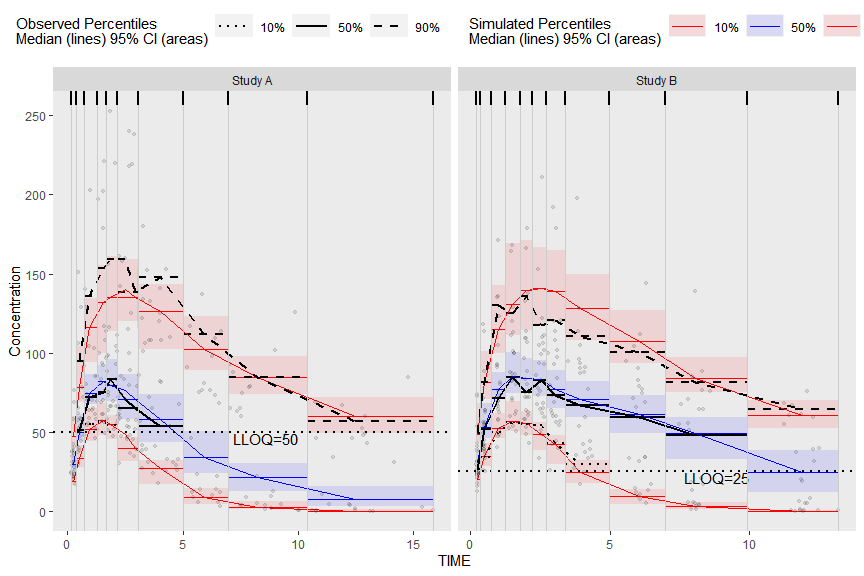Plot Below Quantification Limit (BQL)

If using the censoring() function, the resulting tidyvpcobj will also contain a pctblq table. Use ggplot2 to plot the percentage of data below the limit of quantification across bins.

We can include geom_ribbon() using the lo and hi columns in the vpc$pctblq table to denote the lower/upper bounds of our confidence interval. Let’s also plot the median %blq of the simulated data using the md column in the vpc$pctblq table.

obs_data$LLOQ <- obs_data[, ifelse(STUDY == "Study A", 50, 25)] vpc <- observed(obs_data, x = TIME, y = DV) %>% simulated(sim_data, y = DV) %>% censoring(blq = DV < LLOQ, lloq = LLOQ) %>% stratify(~STUDY) %>% binning(bin = NTIME) %>% vpcstats(qpred = c(0.1, 0.5, 0.9)) ggplot(vpc$pctblq) +
facet_grid(~STUDY) +
geom_ribbon(aes(x = xbin, ymin= lo, ymax = hi), fill = "red", alpha = .2) +
geom_line(aes(x = xbin, y = y)) +
geom_line(aes(x = xbin, y = md), color = "red") +
labs(x= "TIME", y= "% BLQ")